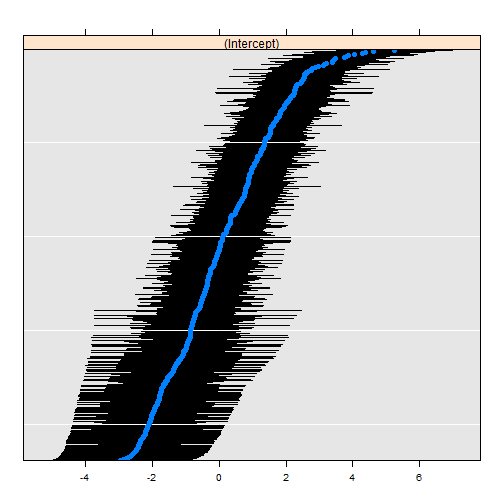# Cancer, Fantasy Football, and Three Level Mixed Effects Logistic Regression

### Just a Little Tweet

This circuitous journey all started with a little tweet.

@ramnath_vaidya @timelyportfolio are there any examples out there that demonstrate how to add error bars to points with rCharts?

— Carson Sievert (@cpsievert) September 7, 2013

I started exploring how `rCharts` could make error bars, and I came up with 3 fine examples courtesy of this tweet

— Christophe Viau (@d3visualization) June 20, 2013

For the original use which I later found out would be fantasy football rankings, I thought this quartile plot would work nicely. I went to work making changes to the quartile plot so that it would play well with `rCharts` and also allow small multiples or facets.

### And Another Little Tweet

Then this morning another tweet.

The UCLA Stats consulting website is a gift that keeps on giving. Great primer on mixed effects logit in #rstats http://t.co/0ZyUNj742i

— hjms (@hjms) September 27, 2013

and at the end of that very good tutorial I found a lattice `dotplot` that when flipped looked remarkably similar to my d3 errorbar.

R code from this fine article

UCLA: Statistical Consulting Group R Data Analysis Examples: Mixed Effects Logistic Regression http://www.ats.ucla.edu/stat/r/dae/melogit.htm Accessed 9/27/2013
``````#require(ggplot2)
#require(GGally)
require(reshape2)
require(lme4)
#require(compiler)
#require(parallel)
#require(boot)

hdp <- within(hdp, {
Married <- factor(Married, levels = 0:1, labels = c("no", "yes"))
DID <- factor(DID)
HID <- factor(HID)
})

# estimate the model and store results in m
m3a <- glmer(remission ~ Age + LengthofStay + FamilyHx + IL6 + CRP +
CancerStage + Experience + (1 | DID) + (1 | HID),
data = hdp, family = binomial, nAGQ=1)

# print the mod results without correlations among fixed effects
# print(m3a, corr=FALSE)

dP <- dotplot(
ranef(m3a, which = "DID", postVar = TRUE),
scales = list(y = list(alternating = 0))
)
print(dP)
``````

\$DIDIt looked so close that I just had to try to replicate it. I scrambled to scrape the surface of Three Level Mixed Effects Logistic Regression just enough to extricate the data needed for the plot. I dug into the `str` of the lattice plot and examined the source code from `lme4`.

This little bit of code gets the data that we will need to plot.

``````## back into the x, y, and errorbar components by looking at
## both the structure of the dotplot and also the lme4 source
## https://github.com/lme4/lme4/blob/bf060a61168499d314b6248da8b2dc468e3af3c9/R/lmer.R#L2190
# str(dP)
## dP\$DID\$panel.args.common gives us the dotplot se which will be our error portion
## looking at the source from lme4 we get by using attr
##    attr(ranef(m3a, which = "DID", postVar = TRUE)\$DID,"postVar")
## dP\$DID\$panel.args\$x or sort(ranef(m3a, which = "DID", postVar = TRUE)\$DID[,1]) will be our y
## dP\$DID\$panel.args\$y or just the index will serve as our x

## now that we know our x, y, and errorbar
## make a data.frame that we will use with rCharts

r <- ranef(m3a, which = "DID", postVar = TRUE)\$DID
dfForPlot <- data.frame(
rownames(r),  #this will be our x
r[,1],            #this will be our y
as.numeric(attr( r, "postVar" ))  #this will be our se
)
colnames(dfForPlot) <- c("id","intercept","se")
``````

### d3-ify in R with rCharts

I never thought I would say this, but the d3/rCharts piece is actually the easiest. Just specify a couple of parameters, and we have an interactive error bar plot.

``````## using very experimental version of rCharts
## require(devtools)
## install_github("rCharts", "timelyportfolio", ref = "test-speedimprove")
require(rCharts)

#set working directory to a local and change setLib and templates\$script
#if going to github gh-pages repo not desired
path = "http://timelyportfolio.github.io/rCharts_errorbar"
#path = getwd()
ePlot <- rCharts\$new()
ePlot\$setLib(path)
ePlot\$templates\$script = paste0(path,"/layouts/chart.html")
#not the way Ramnath intended but we'll hack away
ePlot\$params =  list(
data = dfForPlot,
height = 500,
width = 1000,
margin = list(top = 10, bottom = 10, right = 50, left = 100),
x = "id",
y = "intercept",
sort = list( var = "intercept" ),
whiskers = "#!function(d){return [d.intercept - 1.96 * d.se, d.intercept + 1.96 * d.se]}!#",
tooltipLabels = c("id","intercept","se")
)
ePlot
``````

And since we are spoiled in R by facets in `ggplot2` and strips in `lattice`, I just have to demo the small multiples capability of rCharts after a little bit of tweaking of the js code. I hope the `facet = list(x = "variable")` is simple enough, but soon I think `rCharts` will have a `facet` command to make it even easier.

``````#example of facetting

#let's add the HID to demo facets
r2 <- ranef(m3a, which = "HID", postVar = TRUE)\$HID
#use our data frame dfForPlot as our starting point
dfForFacetPlot <- dfForPlot
dfForFacetPlot\$variable = "DID"
#combine the HID with DID to get long form(molten) data.frame
dfForFacetPlot <- rbind(
dfForFacetPlot,
data.frame(list(
id = rownames(r2),  #this will be our x
intercept = r2[,1],            #this will be our y
se = as.numeric(attr( r2, "postVar" )),  #this will be our se
variable = rep("HID", nrow(r2))
))
)
dfForFacetPlot\$idcolor = floor(as.numeric(levels(dfForFacetPlot\$id)[dfForFacetPlot\$id])/10)

ePlotFacet <- rCharts\$new()
ePlotFacet\$setLib(path)
ePlotFacet\$templates\$script = paste0(path,"/layouts/chart.html")
ePlotFacet\$params =   list(
data = dfForFacetPlot,
height = 500,
width = 1000,
margin = list(top = 10, bottom = 10, right = 50, left = 100),
x = "id",
y = "intercept",
color = "idcolor",
sort = list( var = "intercept" ),
whiskers = "#!function(d){return [d.intercept - 1.96 * d.se, d.intercept + 1.96 * d.se]}!#",
tooltipLabels = c("id","intercept","se"),
facet = list(x = "variable")  #add y for facet grid
)
ePlotFacet
``````

### Thanks

As you can hopefully tell, I depended heavily on lots of folks to write this little post. Thanks to:
1. Ramnath Vaidyanathan - @ramnath_vaidya
2. Carson Sievert - @cpsievert
2. Iain Dillingham
3. UCLA: Statistical Consulting Group
4. `lme4` Team
5. Mike Bostock
6. Everybody else that has contributed R and d3 examples online. I probably have looked at them.